# Test: Co-ordinate Geometry- 3

## 15 Questions MCQ Test Practice Questions for GMAT | Test: Co-ordinate Geometry- 3

Description
Attempt Test: Co-ordinate Geometry- 3 | 15 questions in 15 minutes | Mock test for GMAT preparation | Free important questions MCQ to study Practice Questions for GMAT for GMAT Exam | Download free PDF with solutions
QUESTION: 1

Solution:

QUESTION: 2

### In the rectangular coordinate plane points X and Z lie on the same line through the origin and points W and Y lie on the same line through the origin. If a2 + b2 = c2 + d2 and e2 + f2 = g2 + h2, what is the value of length XZ – length WY?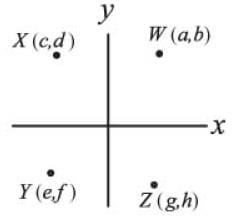Solution:

To find the distance from the origin to any point in the coordinate plane, we take the square root of the sum of the squares of the point's x- and y-coordinates. So, for example, the distance from the origin to point W is the square root of (a2 + b2). This is because the distance from the origin to any point can be thought of as the hypotenuse of a right triangle with legs whose lengths have the same values as the x- and y-coordinates of the point itself: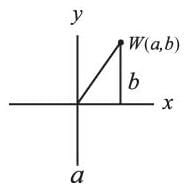We can use the Pythagorean Theorem to determine that a2 + b2 = p2, where p is the length of the hypotenuse from the origin to point W.
We are also told in the question that a2 + b2 = c2 + d2, therefore point X and point W are equidistant from the origin. And since e2 + f2 = g2 + h2, we know that point Y and point Z are also equidistant from the origin.
If the distance from the origin is the same for points W and X, and for points Z and Y, then the length of WY must be the same as the length of XZ. Therefore, the value of length XZ – length WY must be 0.

QUESTION: 3

### In the xy-coordinate system, what is the slope of the line that goes through the origin and is equidistant from the two points P = (1, 11) and Q = (7, 7)?

Solution:

The question asks us to find the slope of the line that goes through the origin and is equidistant from the two points P=(1, 11) and Q=(7, 7). It's given that the origin is one point on the requested line, so if we can find another point known to be on the line we can calculate its slope. Incredibly the midpoint of the line segment between P and Q is also on the requested line, so all we have to do is calculate the midpoint between P and Q! (This proof is given below).

Let's call R the midpoint of the line segment between P and Q. R's coordinates will just be the respective average of P's and Q's coordinates. Therefore R's x-coordinate equals 4 , the average of 1 and 7. Its y-coordinate equals 9, the average of 11 and 7. So R=(4, 9).

Finally, the slope from the (0, 0) to (4, 9) equals 9/4, which equals 2.25 in decimal form.

Proof
To show that the midpoint R is on the line through the origin that's equidistant from two points P and Q, draw a line segment from P to Q and mark R at its midpoint. Since R is the midpoint then PR = RQ.

Now draw a line L that goes through the origin and R.  Finally draw a perpendicular from each of P and Q to the line L. The two triangles so formed are congruent, since they have three equal angles and PR equals RQ. Since the triangles are congruent their perpendicular distances to the line are equal, so line L is equidistant from P and Q.

QUESTION: 4

What is the slope of the line represented by the equation x + 2y = 1?

Solution:

To find the slope of a line, it is helpful to manipulate the equation into slope-intercept form:
y = mx + b, where m equals the slope of the line (incidentally, b represents the y-intercept). After isolating y on the left side of the equation, the x coefficient will tell us the slope of the line.

x + 2y = 1
2y = -x + 1
y = -x/2 + 1/2

The coefficient of x is -1/2, so the slope of the line is -1/2.

QUESTION: 5

A certain square is to be drawn on a coordinate plane. One of the vertices must be on the origin, and the square is to have an area of 100. If all coordinates of the vertices must be integers, how many different ways can this square be drawn?

Solution:

Each side of the square must have a length of 10.  If each side were to be 6, 7, 8, or most other numbers, there could only be four possible squares drawn, because each side, in order to have integer coordinates, would have to be drawn on the x- or y-axis.  What makes a length of 10 different is that it could be the hyptoneuse of a pythagorean triple, meaning the vertices could have integer coordinates without lying on the x- or y-axis.
For example, a square could be drawn with the coordinates (0,0), (6,8), (-2, 14) and (-8, 6).  (It is tedious and unnecessary to figure out all four coordinates for each square).
If we lable the square abcd, with a at the origin and the letters representing points in a clockwise direction, we can get the number of possible squares by figuring out the number of unique ways ab can be drawn.

a has coordinates (0,0) and b could have coordinates:

(-10,0)
(-8,6)
(6,8)
(0,10)
(6,8)
(8,6)
(10,0)
(8, -6)
(6, -8)
(0, 10)
(-6, -8)
(-8, -6)

There are 12 different ways to draw ab, and so there are 12 ways to draw abcd.

QUESTION: 6

Line L contains the points (2,3) and (p,q). If q = 2, which of the following could be the equation of line m, which is perpendicular to line L?

Solution:

First, we determine the slope of line L as follows: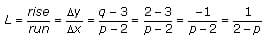If line m is perpendicular to line L, then its slope is the negative reciprocal of line L's slope. (This is true for all perpendicular lines.) Thus:
If the slop of L = a/b , then the slop of m = -a/b
Therefore, the slope of line m can be calculated using the slope of line L as follows: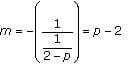This slope can be plugged into the slope-intercept equation of a line to form the equation of line m as follows:
y = (p – 2)x + b
(where (p – 2) is the slope and b is the y-intercept)
This can be rewritten as y = px – 2x + b or 2x + y = px + b as in answer choice A.
An alternative method: Plug in a value for p. For example, let's say that p = 4.
Thus the slop of Line L =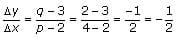The slope of line m is the negative inverse of the slope of line L. Thus, the slope of line m is 2.
Therefore, the correct equation for line m is the answer choice that yields a slope of 2 when the value 4 is plugged in for the variable p.
(A) 2x + y = px + 7   yields   y = 2x + 7
(B) 2x + y = –px   yields   y = –6x
(C) x + 2y = px + 7   yields   y = (3/2)x + 7/2
(D) y – 7 = x ÷ (p – 2)   yields   y = (1/2)x + 7
(E) 2x + y = 7 – px   yields   y = –6x + 7
Only answer choice A yields a slope of 2. Choice A is therefore the correct answer.

QUESTION: 7

The (x, y) coordinates of points P and Q are (-2, 9) and (-7, -3), respectively. The height of equilateral
triangle XYZ is the same as the length of line segment PQ. What is the area of triangle XYZ?

Solution:

The formula for the distance between two points (x1, y1) and (x2, y2) is: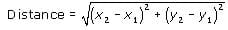One way to understand this formula is to understand that the distance between any two points on the coordinate plane is equal to the hypotenuse of a right triangle whose legs are the difference of the x-values and the difference of the y-values (see figure).  The difference of the x-values of P and Q is 5 and the difference of the y-values is 12.  The hypotenuse must be 13 because these leg values are part of the known right triangle triple: 5, 12, 13.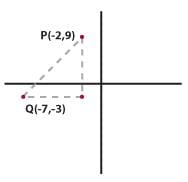We are told that this length (13) is equal to the height of the equilateral triangle XYZ.  An equilateral triangle can be cut into two 30-60-90 triangles, where the height of the equilateral triangle is equal to the long leg of each 30-60-90 triangle. We know that the height of XYZ is 13 so the long leg of each 30-60-90 triangle is equal to 13. Using the ratio of the sides of a 30-60-90 triangle (1:√3:2), we can determine that the length of the short leg of each 30-60-90 triangle is equal to 13/√3. The short leg of each 30-60-90 triangle is equal to half of the base of equilateral triangle XYZ. Thus the base of XYZ =2(13√3) = 26√3
The question asks for the area of XYZ, which is equal to 1/2 × base × height: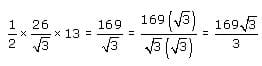QUESTION: 8

The line 3x + 4y = 8 passes through all of the quadrants in the coordinate plane except:

Solution:

If we put the equation 3x + 4y = 8 in the slope-intercept form (y = mx + b), we get:
y = (-3/4)x + 2
This means that m (the slope) = -3/4 and b (the y-intercept) = 2.
We can graph this line by going to the point (0, 2) and going to the right 4 and down 3 to the point (0 + 4, 2 - 3) or (4, -1).
If we connect these two points, (0, 2) and (4, -1), we see that the line passes through quadrants I, II and IV.

QUESTION: 9

The coordinates of points A and C are (0, -3) and (3, 3), respectively. If point B lies on line AC between points A and C, and if AB = 2BC, which of the following represents the coordinates of point B?

Solution:

Point B is on line AC, two-thirds of the way between Point A and Point C. To find the coordinates of point B, it is helpful to imagine that you are a point traveling along line AC.
When you travel all the way from point A to point C, your x-coordinate changes 3 units (from x = 0 to x = 3). Two-thirds of the way there, at point B, your x-coordinate will have changed 2/3 of this amount, i.e. 2 units. The x-coordinate of B is therefore x = 0 + 2 = 2.
When you travel all the way from point A to point C, your y-coordinate changes 6 units (from y = -3 to y = 3). Two-thirds of the way there, at point B, your y-coordinate will have changed 2/3 of this amount, i.e. 4 units. The y-coordinate of B is therefore y = -3 + 4 = 1.
Thus, the coordinates of point B are (2,1).

QUESTION: 10

In the xy-coordinate system, rectangle ABCD is inscribed within a circle having the equation x2 + y2 = 25. Line segment AC is a diagonal of the rectangle and lies on the x-axis. Vertex B lies in quadrant II and vertex D lies in quadrant IV. If side BC lies on line y = 3x + 15, what is the area of rectangle ABCD?

Solution:

The equation of a circle given in the form  x2 + y2 = r2 indicates that the circle has a radius of r and that its center is at the origin (0,0) of the xy-coordinate system.  Therefore, we know that the circle with the equation  x2 + y2 = 25 will have a radius of 5 and its center at (0,0).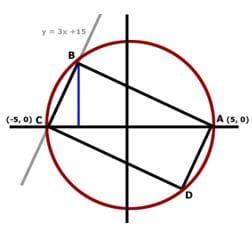If a rectangle is inscribed in a circle, the diameter of the circle must be a diagonal of the rectangle (if you try inscribing a rectangle in a circle, you will see that it is impossible to do so unless the diagonal of the rectangle is the diameter of the circle).  So diagonal AC of rectangle ABCD is the diameter of the circle and must have length 10 (remember, the radius of the circle is 5).  It also cuts the rectangle into two right triangles of equal area.  If we find the area of one of these triangles and multiply it by 2, we can find the area of the whole rectangle.

We could calculate the area of right triangle ABC if we had the base and height.  We already know that the base of the triangle, AC, has length 10.  So we need to find the height.

The height will be the distance from the x-axis to vertex B.  We need to find the coordinate of point B in order to find the height.  Since the circle intersects triangle ABCD at point B, the coordinates of point B will satisfy the equation of the circle x2 + y2 = 25. Point B also lies on the line y=3x+15, so the coordinates of point B will satisfy that equation as well.
Since the values of x and y are the same in both equations and since  y=3x+15, we can substitute (3x + 15) for y in the equation x2 + y2 = 25 and solve for x: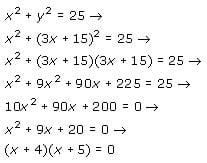So the two possible values of x are -4 and -5.  Therefore, the two points where the circle and line intersect (points B and C) have x-coordinates -4 and -5, respectively.  Since the x-coordinate of point C is -5 (it has coordinates (-5, 0)), the x-coordinate of point B must be -4.  We can plug this into the equation   and solve for the y-coordinate of point B:
y=3(-4) +15 →
y=-12+15→
y=3
So the coordinates of point B are (-4, 3) and the distance from the x-axis to point B is 3, making the height of triangle ABC equal to 3.  We can now find the area of triangle ABC: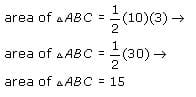The area of rectangle ABCD will be twice the area of triangle ABC.  So if the area of triangle ABC is 15, the area of rectangle ABCD is (2)(15) = 30.

QUESTION: 11

The line represented by the equation y = 4 – 2x is the perpendicular bisector of line segment RP. If R has the coordinates (4, 1), what are the coordinates of point P?​

Solution:

First, rewrite the line y=4-2x as y=-2x+4 . The equation is now in the form y=mx+b where m represents the slope and b represents the y-intercept. Thus, the slope of this line is -2.
By definition, if line F is the perpendicular bisector of line G, the slope of line F is the negative inverse of the slope of line G. Since we are told that the line
y=-2x+4 is the perpendicular bisector of line segment RP, line segment RP must have a slope of 1/2 (which is the negative inverse of ).
Now we know that the slope of the line containing segment RP is 1/2 but we do not know its y-intercept. We can write the equation of this line as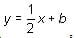where b represents the unknown y-intercept.

To solve for b, we can use the given information that the coordinates of point R are (4, 1). Since point R is on the line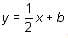we can plug 4 in for x and 1 in for y as follows: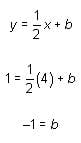Now we have a complete equation for the line containing segment RP: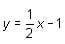We also have the equation of the perpendicular bisector of this line: y=-2x+4. To determine the point M at which these two lines intersect, we can set these two equations to equal each other as follows: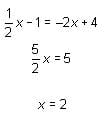Thus, the intersection point M has x-coordinate 2. Using this value, we can find the y coordinate of point M:
y=-2x+4
y=-2(2)+4
y=0
Thus the perpendicular bisector intersects line segment RP at point M, which has the coordinates (2, 0). Since point M is on the bisector of RP, point M represents the midpoint on line segment RP; this means that it is equidistant from point R and point P.
We know point R has an x-coordinate of 4. This is two units away from the x-coordinate of midpoint M, 2. Therefore the x-coordinate of point P must also be two units away from 2, which is 0.
We know point R has a y-coordinate of 1. This is one unit away from the y-coordinate of midpoint M, 0. Therefore, the y-coordinate of point P must also be one unit away from 0, which is –1.
The coordinates of point P are
(0,-1). The correct answer is D.

QUESTION: 12

A certain computer program randomly generates equations of lines in the form y = mx + b. If point P
is a point on a line generated by this program, what is the probability that the line does NOT pass through figure ABCD?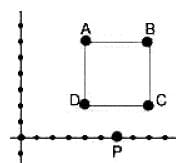Solution: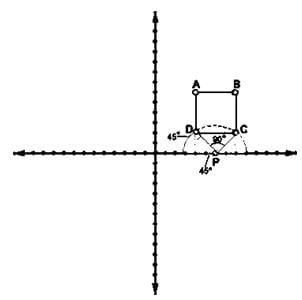The most difficult part of this question is conceptualizing what you're asked to find. The best way to handle tricky problems like this is to break them down into their component parts, resolve each part, then reconstruct them as a whole. You start off with a coordinate plane and four points (A, B, C, and D) that form a square when joined. Beneath the square, on the x-axis, lies another point, P. Then you are asked to determine the probability that a line randomly drawn through P will not also pass through square ABCD. In any probability question, the first thing you need to determine is the number of total possibilities. In this case, the total number of possibilities will be the total number of lines that can be drawn through P. The problem, though, is that there are literally infinitely many lines that satisfy this criterion. We cannot use infinity as the denominator of our probability fraction. So what to do?
This is where some creative thinking is necessary. First, you need to recognize that there must be a limited range of possibilities for lines that pass through both P and ABCD. If that were not the case, the probability would necessarily be 1 or 0. What is this range? Well, drawing out a diagram of the problem will help enormously here. Any line that passes through both P and ABCD has to pass through a triangle formed by ABP. This triangle is an isosceles right triangle. We know this because if we drop perpendiculars from points A and B, we end up with two isosceles right triangles, with angles of 45 degrees on either side of P. Since angles along a straight line must equal 180, we know that angle APB must measure 90 degrees. So if angle APB measures 90 degrees, we can use that to figure out the proportion of lines that pass through both P and ABCD. If we draw a semicircle with P as its center, we can see that the proportion of lines passing through P and ABCD will be the same as the proportion of the semicircle occupied by sector APB. Since a semicircle contains 180 degrees, sector APB occupies 1/2 of the semicircle. So 1/2 of all possible lines through P will also pass though ABCD, which means the 1/2 will NOT pass through ABCD and we have our answer.

QUESTION: 13

In the rectangular coordinate system, a line passes through the points (0,5) and (7,0). Which of the following points must the line also pass through?

Solution:

Because we are given two points, we can determine the equation of the line. First, we'll calculate the slope by using the formula (y2 – y1) / (x2 – x1):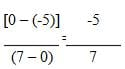Because we know the line passes through (0,5) we have our y-intercept which is 5.
Putting these two pieces of information into the slope-intercept equation gives us y = (-5/7)x + 5. Now all we have to do is plug in the x-coordinate of each of the answer choices and see which one gives us the y-coordinate.
(A) (-14, 10)
y = -5/7(-14) + 5 = 15; this does not match the given y-coordinate.
(B) (-7, 5)
y = -5/7(-7) + 5 = 10; this does not match the given y-coordinate.
(C) (12, -4)
y = -5/7(12) + 5 = -60/7 + 5, which will not equal an integer; this does not match the given y-coordinate.
(D) (-14, -5)
y = -5/7(-14) + 5 = -5; this matches the given y-coordinate so we have found our answer.
(E) (21, -9)
y = -5/7(21) + 5 = -15/7 + 5, which will not equal an integer; this does not match the given y-coordinate.  (Note that you do not have to test this answer choice if you've already discovered that D works.)

QUESTION: 14

Which of the following equations represents a line that is perpendicular to the line described by the equation 3x + 4y = 8?

Solution:

If we put the equation 3x + 4y = 8 in the slope-intercept form (y = mx + b), we get: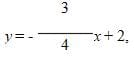which means that m (the slope)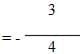. The slope of a line perpendicular to this line must be  4/3, the negative reciprocal of  -3/4.
Among the answer choices, only E gives an equation with a slope of 4/3.

QUESTION: 15

How many units long is the straight line segment that connects the points (-1,1) and (2,6) on a rectangular coordinate plane?

Solution:

We are essentially asked to find the distance between two points.  The simplest method is to sketch a coordinate plane and draw a right triangle using the two given points: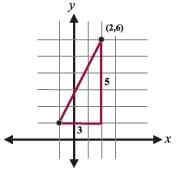We can now see that one leg of the triangle is 3 and the other leg is 5.  Because it is a right triangle, we can use the Pythagorean Theorem to calculate the hypotenuse, which is the line segment whose length we are asked to calculate.
32 + 52 = c2
34 = c2
c= √34

Note: always be careful!  Some people will notice the lengths 3 and 5 and automatically assume this is a 3-4-5 right triangle.  This one is not, however, because the hypotenuse must always be the longest side and, in this problem, the leg is 5 units long, not the hypotenuse.Use Code STAYHOME200 and get INR 200 additional OFF Use Coupon Code